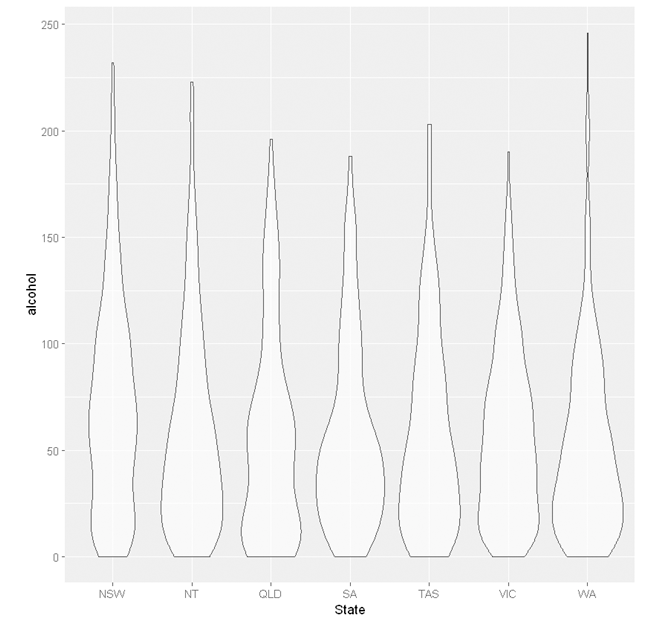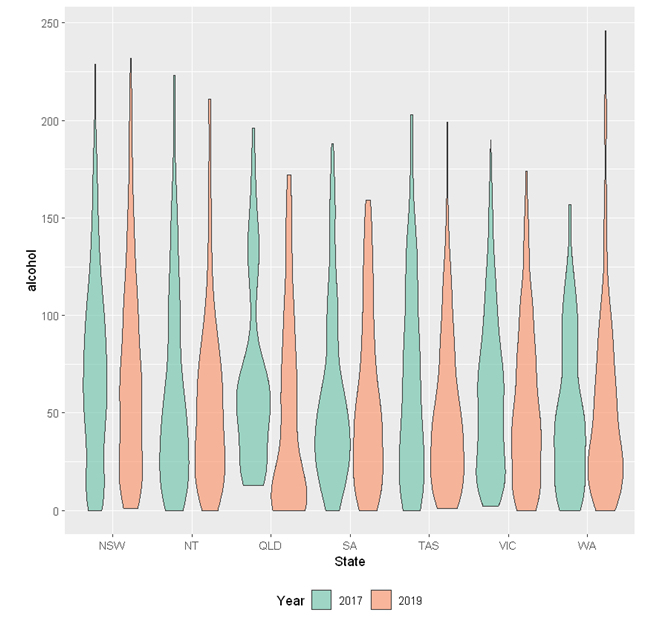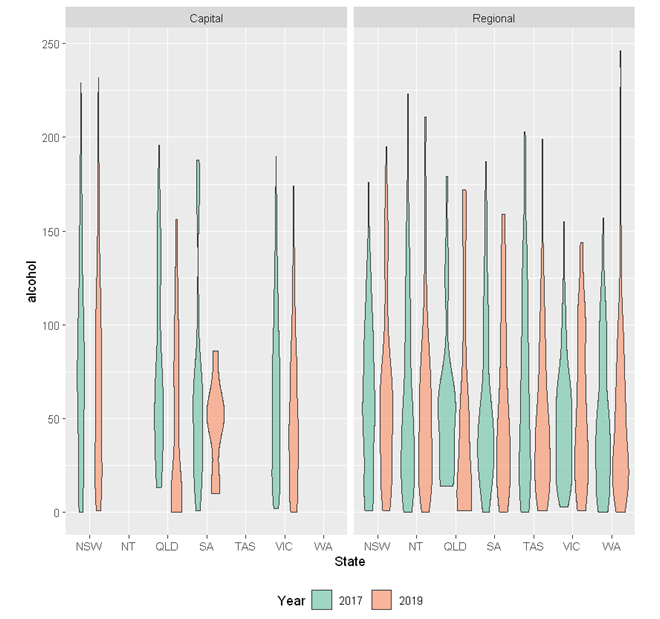# StatsNotebook

## Violin plot

20 Sep, 2020 | 4 minutes read

Tags: DataViz, R code

The tutorial is based on R and StatsNotebook, a graphical interface for R.

Violin plot can be used to visualise the distribution of a numeric variable across several groups. StatsNotebook uses `geom_violin()` from `ggplot2` to build violin plot.

We use the built-in alcohol dataset in this example. This dataset can be loaded into StatsNotebook using instruction here. This is a simulated data of alcohol consumption from 3666 individuals.

This dataset can also be loaded using the following codes

``````library(tidyverse)
``````

We will use the following three variables from this dataset

1. alcohol - Number of standard drinks consumed in a month
2. Remoteness - Capital city or regional area
3. State - Seven states/territories in Australia: Queensland, New South Wales, Northern Territory, South Australia, Tasmania, Victoria and Western Australia.

In this example, we will build

1. a violin plot comparing distributions by one grouping variable
2. a violin plot comparing distributions by two grouping variables
3. multiple violin plots in different facets

To build a violin plot for a single numeric variable (e.g. alcohol) by groups (e.g. State),

1. Click DataViz at the top
2. Click Numeric
3. Select Boxplot/ Violin plot from the menu
4. In the Boxplot/ violin plot panel, select alcohol to Vertical axis and select State to Horizontal aixs.
5. Expand Boxplot/ Violin plot Setting panel, check Violin plot.
6. Click Code and Run.
``````currentDataset %>%
drop_na(State) %>%
ggplot(aes(y = alcohol, x = State)) +
geom_violin(alpha = 0.6, na.rm=TRUE)+
scale_fill_brewer(palette = "Set2")+
scale_color_brewer(palette = "Set2")+
theme(legend.position = "bottom")

"Chan, G. and StatsNotebook Team (2020). StatsNotebook. (Version 0.1.0) [Computer Software]. Retrieved from https://www.statsnotebook.io"
"R Core Team (2020). The R Project for Statistical Computing. [Computer software]. Retrieved from https://r-project.org"
"Wickham H (2016). ggplot2: Elegant Graphics for Data Analysis. Springer-Verlag New York. ISBN 978-3-319-24277-4, https://ggplot2.tidyverse.org"
``````To build a violin plot for a single numeric variable (e.g. alcohol) by two grouping variables (e.g. State and Year),

1. Click DataViz at the top
2. Click Numeric
3. Select Boxplot/ Violin plot from the menu
4. In the boxplot/ violin plot panel, select alcohol to Vertical axis, select State to Horizontal aixs and Year to Split by Fill color.
5. Expand Boxplot/ Violin plot Setting panel, check Violin plot.
6. Click Code and Run.
``````currentDataset %>%
drop_na(State, Year) %>%
ggplot(aes(y = alcohol, x = State, fill = Year)) +
geom_violin(alpha = 0.6, na.rm=TRUE)+
scale_fill_brewer(palette = "Set2")+
scale_color_brewer(palette = "Set2")+
theme(legend.position = "bottom")

"Chan, G. and StatsNotebook Team (2020). StatsNotebook. (Version 0.1.0) [Computer Software]. Retrieved from https://www.statsnotebook.io"
"R Core Team (2020). The R Project for Statistical Computing. [Computer software]. Retrieved from https://r-project.org"
"Wickham H (2016). ggplot2: Elegant Graphics for Data Analysis. Springer-Verlag New York. ISBN 978-3-319-24277-4, https://ggplot2.tidyverse.org"

``````To build multiple violin plot for a single numeric variable (e.g. alcohol) by groups (e.g. States abd Year) in multiple facets (by another variable, e.g. Remoteness),

1. Click DataViz at the top
2. Click Numeric
3. Select Boxplot/ Violin plot from the menu
4. In the Boxplot/ violin plot panel, select alcohol to Vertical axis, State to Horizontal axis, Year to Split by: Fill Color and Remoteness to Facet.
5. Expand Boxplot/ Violin plot Setting panel, check Violin plot.
6. Click Code and Run.
``````currentDataset %>%
drop_na(State, Year, Remoteness) %>%
ggplot(aes(y = alcohol, x = State, fill = Year)) +
geom_violin(alpha = 0.6, na.rm=TRUE)+
scale_fill_brewer(palette = "Set2")+
scale_color_brewer(palette = "Set2")+
facet_wrap( ~ Remoteness)+
theme(legend.position = "bottom")

"Chan, G. and StatsNotebook Team (2020). StatsNotebook. (Version 0.1.0) [Computer Software]. Retrieved from https://www.statsnotebook.io"
"R Core Team (2020). The R Project for Statistical Computing. [Computer software]. Retrieved from https://r-project.org"
"Wickham H (2016). ggplot2: Elegant Graphics for Data Analysis. Springer-Verlag New York. ISBN 978-3-319-24277-4, https://ggplot2.tidyverse.org"
````````````Chan, G. and StatsNotebook Team (2020). StatsNotebook. [Computer Software]. Retrieved from https://www.statsnotebook.io
R Core Team (2020). The R Project for Statistical Computing. [Computer software]. Retrieved from https://r-project.org
Wickham H (2016). ggplot2: Elegant Graphics for Data Analysis. Springer-Verlag New York. ISBN 978-3-319-24277-4, https://ggplot2.tidyverse.org
``````